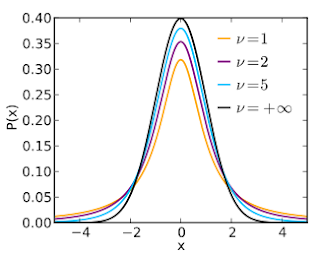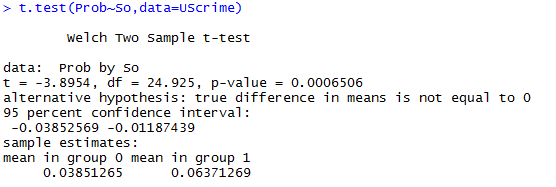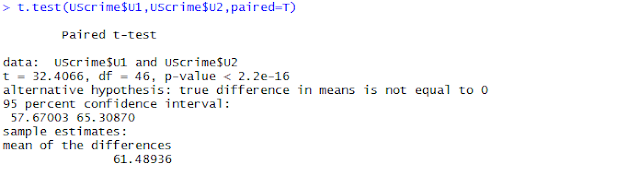## Schedule an e-mail delivery for your existing reports in Google Data Studio

In this post you will learn about, how you can schedule an e-mail delivery of your Data Studio dashboards. This is a recent addition to the Data Studio arsenal making it one notch higher when it comes to data visualization

With this new added feature to the data studio you can easily send the PDF format of the report by just scheduling the e-mail delivery in few simple steps. This will save you a lot of time and improve accessibility of your beautiful dashboards with stakeholders at multiple levels in your organization

So, how can you schedule an e-mail delivery for your reports?

1. View the report you want to schedule.

2. Click on the option “Schedule email delivery” in the upper right corner.

3. Select the date and time you want for the delivery of report to recipients.

4. Set the delivery frequency for the report.

Enjoy coffee.

If you need help with this, then we are a crazy team of Google and Adobe Certified Analytics Experts who eat, breathe, sleep and dream Analytics. And we’ve made it our purpose and passion to electrify your business’s potential through Analytics.

Found it informative? Leave a comment! You can also give us a thumbs up by sharing it with your community. Also did you know that you can light up our day by subscribing to our blog? Subscribe here –

## What is t-test? How to do t-test in R?

Alright, first thing that makes me curious about Student’s t-test is why it is called so. The history is somewhat interesting.

First, why the “Student”?

“Student” is actually pseudonym or pen name of the author, Mr. William Sealy Gosset. He used a pen name because at the time he came up the concept he was not allowed to publish stuff under his own name by his employer. Some company with bad GTPW ratings it seems

Here is the dude –Second, why the “T”?

“t-test” comes from “t-distribution” which in turn comes from “t-statistic” which in turn stands for “test – statistic” which in turn is short for “hypothesis test statistic”

So, brother, what is hypothesis test statistic man?

Hint1: It is used to test some hypothesis!

Hint2: hypothesis to verify any existence of difference between two data sets!

Yes, you smart ass, you got it right! t-statistic is used to perform hypothesis testing around sample mean. But, wait a second. There are plethora of methods out there to test hypothesis around sample means, but when will ya prefer t-test?

Good Question!

The fundamental requirement for using the t-test is that your population should be normally distributed. If you are not sure about the distribution of the population, you can still use the t-distribution if your sample is large enough to satisfy the central limit theorem. If the case is not so, go for some other test like Mann-Whittney U-test.

Second, you will use t-test when the standard deviation of the “Population” is not known. There are like thousands of posts out there who claim that t-statistic should be used when the sample size is less than equal to 30. But, this is not true. So, reiterating : Use a t-test when the standard deviation of the population is not know.

Now, what is t-statistic?

Expressing it in words:

Lets understand t-statistic for a small sample of variable X. The mean of the population is M and mean of sample is Ms.

t-statistic is a ratio of variance between groups to variance within the groups.

So, the formula –

t-statistic = (Ms – M)/SE

Moving forward, lets understand the t-distribution –

Now, as we can see above t-statistic is dependent on standard error, which in turn is dependent on the size of the sample. And because different samples will have different standard errors and different degrees of freedom, there will be multiple t-distributions of the sample variable based on the size of the sample.

How does t-distribution look?

t-distribution is also a bell shaped curve, but it has more area in the tails as compared to area in the tails of normal random distribution of a population. As the size of the sample increases the shape of t-distribution evolves to match the shape of the normal random population distribution and at a sample size of infinity, t-distribution is exactly like the normal random population distribution.Now, how do you do a t-test?

Any statistical test will have some confidence interval. For a given confidence interval and degree of freedom, you will use the t-statistic to calculate the p-value using which you will accept or reject the null hypothesis.

p-value on a t-distribution curve for a particular t-statistic will be area of the curve to the right of that t-statistic

The degree of freedom for a sample of size n is n-1.

Before explaining the steps, let me caress upon one tail/ two tail tests. By default all tests are two tail, which assumes that your sample mean can either be less or more than the population mean, that is you test in both the directions, that’s why 2 tail.

So, the null hypothesis of a two tail test is that there is no difference between sample mean and population mean.

When you take a single tail test, then depending upon whether it is upper tail or lower tail, you check for only one case. If its lower tail, you test whether the sample mean is less than or equal to population mean or not. And if it is upper tail, you test whether the sample mean is greater than or equal to the population mean or not.

So, the null hypothesis of a one tail test, based on the choice of tail, would be either the sample mean being greater than population mean or it being less than the population mean.

Alrighty!

The complement of confidence interval is level of significance. For example, if you have a 95% confidence interval then you level of significance is 5%. Now, depending on whether you take one tail or two tail test, you will use the significance level.

Just to clarify what a 5% level of significance indicates – You can erroneously reject the null hypothesis in probably 5% cases. That is 95% of the times you will not make an error.

Now, finally, the steps –

Case #1 – H0: Sample mean is more than population mean

1. Calculate the t-statistic using the formula

2. For a given t-statistic using software find out the corresponding p value

As mentioned p value gives area to the right of the test statistic on t-distribution curve. That area corresponds to probability of type 1 error

3. Compare p value with level of significance

4. if p value is less than level of significance reject the null hypothesis

Case #2 – Sample mean is less than population mean

Step 1 and 2 are same as above

3. Subtract the p-value from 1

This gives the area to the left of t-statistic on the t-distribution curve

Case # 3 – Sample mean is not equal to population mean, it can be either greater or lesser

Step 1 and 2 are same

3. Double the p-value to accommodate both the cases

4. Compare the doubled value with level of significance

Easy peasy!

Now, ladies and gentlemen lets see how to do a t-test in “R”

Lets start with the independent t-test. Independent t-test is used to prove/disprove similarity between samples of data taken from independent populations.

To understand this, lets the “UScrime” data set in the “MASS” packageHere, the variable SO tells whether the data point is for southern state or not. Prob tells about the probability of imprisonment.

We want to find whether the probability of imprisonment any way affected by the state.

Our Null Hypothesis is that there is no impact on imprisonment based on the state.

The function to run the t-test in R is t.test. It takes either of the following syntax :

t.test(y~x,data)

where x is a dichotomous variable and y is numeric

or

t.test(y1,y2)

where y1 and y2 both are numeric

By default a 2-tail test is assumed. If you want to make it a one tail, then you need to add another argument – alternative=”less” or alternative=”greater”

Lets run the test –Here the p-value is very less than the significance level of 0.05. That is there is indeed a difference between the data from South and non south states. And the probability of this difference being just because of chance is less than 5%.

Next, Dependent t-tests

In case of dependent t-tests, the two populations are not independent of each other, that is they affect each other. Lets take case of unemployment, for limited number of jobs, employment of younger people will affect the employment of some what older people and vice versa

To run a dependent t test in R we simply need to add “paired = T” argument along with the data inputs in the t.test() function.

t.test(y1,y2,paired=T)

where y1 and y2 are numeric data

Ex-With this more or less we should be good with t-test.

Best,

Sanmeet

Founder, DataVinci[testimonials_cycle theme=”default_style” count=”-1″ order_by=”date” order=”ASC” show_title=”0″ use_excerpt=”0″ show_thumbs=”1″ show_date=”1″ show_other=”1″ hide_view_more=”0″ output_schema_markup=”1″ show_rating=”stars” testimonials_per_slide=”1″ transition=”fade” timer=”5000″ pause_on_hover=”true” auto_height=”container” show_pager_icons=”1″ prev_next=”1″ display_pagers_above=”0″ paused=”0″]